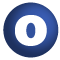# Sorting

#### object Sorting extends AnyRef

The Sorting object provides functions that can sort various kinds of objects. You can provide a comparison function, or you can request a sort of items that are viewable as `Ordered`. Some sorts that operate directly on a subset of value types are also provided. These implementations are derived from those in the Sun JDK.

Note that stability doesn't matter for value types, so use the quickSort variants for those. `stableSort` is intended to be used with objects when the prior ordering should be preserved, where possible.

1. AnyRef
2. Any

### Value Members

1. #### def equals(arg0: Any): Boolean

This method is used to compare the receiver object (`this`) with the argument object (`arg0`) for equivalence

This method is used to compare the receiver object (`this`) with the argument object (`arg0`) for equivalence.

The default implementations of this method is an equivalence relation:

• It is reflexive: for any instance `x` of type `Any`, `x.equals(x)` should return `true`.
• It is symmetric: for any instances `x` and `y` of type `Any`, `x.equals(y)` should return `true` if and only if `y.equals(x)` returns `true`.
• It is transitive: for any instances `x`, `y`, and `z` of type `AnyRef` if `x.equals(y)` returns `true` and `y.equals(z)` returns `true`, then `x.equals(z)` should return `true`.

If you override this method, you should verify that your implementation remains an equivalence relation. Additionally, when overriding this method it is often necessary to override `hashCode` to ensure that objects that are "equal" (`o1.equals(o2)` returns `true`) hash to the same `Int` (`o1.hashCode.equals(o2.hashCode)`).

arg0

the object to compare against this object for equality.

returns

`true` if the receiver object is equivalent to the argument; `false` otherwise.

definition classes: AnyRef ⇐ Any
2. #### def hashCode(): Int

Returns a hash code value for the object

Returns a hash code value for the object.

The default hashing algorithm is platform dependent.

Note that it is allowed for two objects to have identical hash codes (`o1.hashCode.equals(o2.hashCode)`) yet not be equal (`o1.equals(o2)` returns `false`). A degenerate implementation could always return `0`. However, it is required that if two objects are equal (`o1.equals(o2)` returns `true`) that they have identical hash codes (`o1.hashCode.equals(o2.hashCode)`). Therefore, when overriding this method, be sure to verify that the behavior is consistent with the `equals` method.

definition classes: AnyRef ⇐ Any

4. #### def quickSort(a: Array[Float]): Unit

Quickly sort an array of Floats

Quickly sort an array of Floats.

5. #### def quickSort(a: Array[Int]): Unit

Quickly sort an array of Ints

Quickly sort an array of Ints.

6. #### def quickSort[K](a: Array[K])(arg0: (K) ⇒ Ordered[K]): Unit

Quickly sort an array of items that are viewable as ordered

Quickly sort an array of items that are viewable as ordered.

7. #### def quickSort(a: Array[Double]): Unit

Quickly sort an array of Doubles

Quickly sort an array of Doubles.

8. #### def seq2RichSort[K <: Ordered[K]](s: Seq[K])(arg0: ClassManifest[K]): RichSorting[K]

Provides implicit access to sorting on arbitrary sequences of orderable items. This doesn't quite work the way that I want yet -- K should be bounded as viewable, but the compiler rejects that.

attributes: implicit
9. #### def stableSort[K, M](a: Seq[K], f: (K) ⇒ M)(arg0: ClassManifest[K], arg1: (M) ⇒ Ordered[M]): Array[K]

Stably sorts a sequence of items given an extraction function that will return an ordered key from an item

Stably sorts a sequence of items given an extraction function that will return an ordered key from an item.

a

the sequence to be sorted.

f

the comparison function.

returns

the sorted sequence of items.

10. #### def stableSort[K](a: Seq[K])(arg0: (K) ⇒ Ordered[K], arg1: ClassManifest[K]): Array[K]

Sorts an arbitrary sequence of items that are viewable as ordered

Sorts an arbitrary sequence of items that are viewable as ordered.

11. #### def stableSort[K](a: Seq[K], f: (K, K) ⇒ Boolean)(arg0: ClassManifest[K]): Array[K]

Sorts an arbitrary sequence into an array, given a comparison function that should return `true` iff parameter one is strictly less than parameter two

Sorts an arbitrary sequence into an array, given a comparison function that should return `true` iff parameter one is strictly less than parameter two.

a

the sequence to be sorted.

f

the comparison function.

returns

the sorted sequence of items.

12. #### def stableSort[K](a: Array[K], f: (K, K) ⇒ Boolean)(arg0: ClassManifest[K]): Unit

Sorts an array of `K` given an ordering function `f`

Sorts an array of `K` given an ordering function `f`. `f` should return `true` iff its first parameter is strictly less than its second parameter.

13. #### def stableSort[K](a: Array[K])(arg0: (K) ⇒ Ordered[K], arg1: ClassManifest[K]): Unit

Sort an array of K where K is Ordered, preserving the existing order where the values are equal

Sort an array of K where K is Ordered, preserving the existing order where the values are equal.

14. #### def toString(): String

Returns a string representation of the object

Returns a string representation of the object.

The default representation is platform dependent.

definition classes: AnyRef ⇐ Any